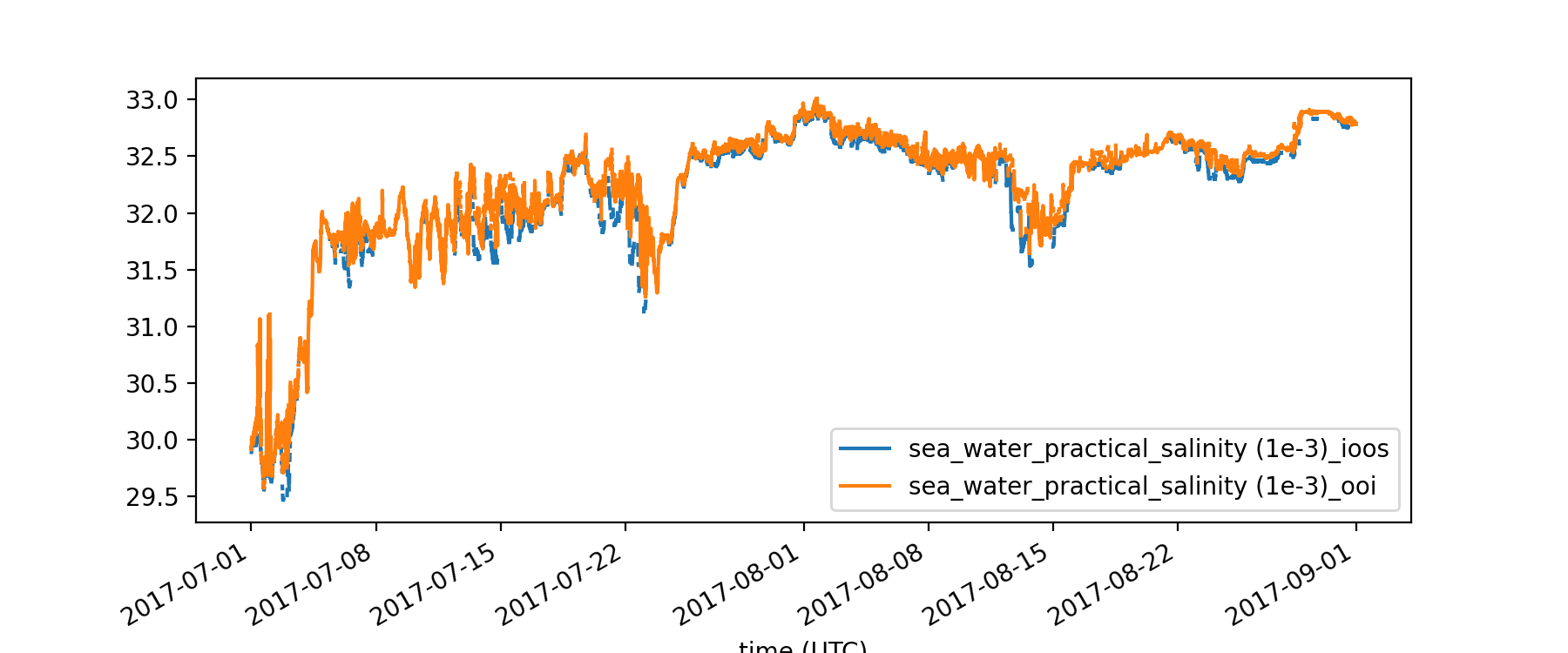# Searching multiple ERDDAP servers#

Created: 2021-10-19

The latest erddapy module release (v1.2.0) added a multiple servers search similar to the web one implemented in https://mathewbiddle.github.io/search-erddaps. The Python interface allow the user to mix powerful variable handling and visualization with the query results.

Let us explore an example based on this Rich Signell’s gist where we search for salinity time-series data in a specific region and time span.

min_time = "2017-07-01T00:00:00Z"
max_time = "2017-09-01T00:00:00Z"
min_lon, max_lon = -127, -123.75
min_lat, max_lat = 43, 48
standard_name = "sea_water_practical_salinity"

kw = {
"standard_name": standard_name,
"min_lon": min_lon,
"max_lon": max_lon,
"min_lat": min_lat,
"max_lat": max_lat,
"min_time": min_time,
"max_time": max_time,
"cdm_data_type": "timeseries",
}

servers = {
"ooi": "https://erddap.dataexplorer.oceanobservatories.org/erddap/",
"ioos": "https://erddap.sensors.ioos.us/erddap/",
}


We will restrict the search to two servers, IOOS sensors and OOI Data Explorer. If one leaves the server_list empty the search will iterate all servers listed in the Awesome ERDDAP list.

from erddapy.multiple_server_search import advanced_search_servers


Title Institution Dataset ID Server url
0 Coastal Endurance: Oregon Inshore Surface Moor... Ocean Observatories Initiative (OOI) ooi-ce01issm-rid16-02-flortd000 https://erddap.dataexplorer.oceanobservatories...
1 Coastal Endurance: Oregon Inshore Surface Moor... Ocean Observatories Initiative (OOI) ooi-ce01issm-rid16-03-ctdbpc000 https://erddap.dataexplorer.oceanobservatories...
2 Coastal Endurance: Oregon Inshore Surface Moor... Ocean Observatories Initiative (OOI) ooi-ce01issm-rid16-03-dostad000 https://erddap.dataexplorer.oceanobservatories...
3 Coastal Endurance: Oregon Inshore Surface Moor... Ocean Observatories Initiative (OOI) ooi-ce01issm-rid16-07-nutnrb000 https://erddap.dataexplorer.oceanobservatories...
4 Coastal Endurance: Oregon Inshore Surface Moor... Ocean Observatories Initiative (OOI) ooi-ce01issm-rid16-06-phsend000 https://erddap.dataexplorer.oceanobservatories...

Now that we have a list of dataset_ids we can iterate and get their positions to create a map. The functions below will create a download URL for the longitude and latitude and request the data as a pandas dataframe.

import pandas as pd
from erddapy import ERDDAP

kw = {
"longitude>=": min_lon,
"longitude<=": max_lon,
"latitude>=": min_lat,
"latitude<=": max_lat,
"time>=": min_time,
"time<=": max_time,
}

e = ERDDAP(server, protocol="tabledap")
dataset_id=dataset_id,
response="csvp",
variables=["longitude", "latitude"],
constraints=kw,
distinct=True,
)
return url

return df.values.squeeze().tolist()


Now we can iterate all the dataset ids we found. That is a slow operation depending on the number of datasets and it is good practice to save/cache the results to re-use them later. That will not only save us some time but it is also appreciated by the data providers maintaining the servers.

import pickle
from pathlib import Path
from urllib.error import HTTPError

import numpy as np
from tqdm.notebook import tqdm

path = Path("positions.p")

if not path.exists():
positions = []
for idx, row in tqdm(df.iterrows()):
try:
pos = request_positions(url)
except HTTPError:
pos = [np.NaN, np.NaN]
positions.append(pos)
with open(path, "wb") as f:
pickle.dump(positions, f)
else:
with open(path, "rb") as f:

df.loc[:, "lon"] = np.array(positions)[:, 0]
df.loc[:, "lat"] = np.array(positions)[:, 1]

df.dropna(inplace=True)

df_ioos = df.loc[df["Server url"] == servers["ioos"]]
df_ooi = df.loc[df["Server url"] == servers["ooi"]]

from ipyleaflet import (
AwesomeIcon,
FullScreenControl,
LegendControl,
Map,
Marker,
Rectangle,
)
from ipywidgets import HTML

colors = {
"IOOS": "blue",
"OOI": "orange",
}

legend = LegendControl(
colors,
name="Dataset locations",
position="bottomright",
)

def make_popup(row):
classes = "table table-striped table-hover table-condensed table-responsive"
return pd.DataFrame(row[["Institution", "Dataset ID"]]).to_html(classes=classes)

m = Map(center=((min_lat + max_lat) / 2, (min_lon + max_lon) / 2), zoom=6)

rectangle = Rectangle(
bounds=((min_lat, min_lon), (max_lat, max_lon)),
color="red",
fill=False,
)

# IOOS
for k, row in df_ioos.iterrows():
marker = Marker(
icon=AwesomeIcon(name="life-ring", marker_color=colors["IOOS"]),
location=(row["lat"], row["lon"]),
)
msg = HTML()
msg.value = make_popup(row)
marker.popup = msg

# OOI
for k, row in df_ooi.iterrows():
marker = Marker(
icon=AwesomeIcon(name="life-ring", marker_color=colors["OOI"]),
location=(row["lat"], row["lon"]),
)
msg = HTML()
msg.value = make_popup(row)
marker.popup = msg

m


User may also look for data that are located near to each other for sensor comparison or calibration. The nested loop below is a simple way to find the stations that are nearest to each other starting from a 1 km distance.

import seawater as sw

dist = 1e3
for k_i, (lon_i, lat_i) in df_ioos[["lon", "lat"]].iterrows():
for k_o, (lon_o, lat_o) in df_ooi[["lon", "lat"]].iterrows():
lats = lat_i, lat_o
lons = lon_i, lon_o
new_dist, angle = sw.dist(lats, lons)
if new_dist < dist:
dist = new_dist
ki, ko = k_i, k_o

print(f"The stations closest ({dist.squeeze():.2f} km) to each other are:")

The stations closest (0.65 km) to each other are:

df.loc[ki]

Title                                46098 - Moored Buoy
Institution    Observing System Monitoring Center (OSMC)
Dataset ID                                    osmc_46098
Server url        https://erddap.sensors.ioos.us/erddap/
lon                                              -124.95
lat                                            44.383335
Name: 65, dtype: object

df.loc[ko]

Title          Coastal Endurance: Oregon Offshore Surface Moo...
Institution                 Ocean Observatories Initiative (OOI)
Dataset ID                       ooi-ce04ossm-rid27-02-flortd000
Server url     https://erddap.dataexplorer.oceanobservatories...
lon                                                   -124.94508
lat                                                     44.37868
Name: 15, dtype: object

def download_salinity(server, dataset_id):
e = ERDDAP(server, protocol="tabledap")
e.dataset_id = dataset_id
e.constraints = {"time>=": min_time, "time<=": max_time}
e.response = "csv"
e.variables = [
"time",
e.get_var_by_attr(dataset_id=dataset_id, standard_name=standard_name),
]

col = "time (UTC)"
return e.to_pandas(parse_dates=[col], index_col=col)

dataset_id = df.loc[ki]["Dataset ID"]


Downloading dataset_id='osmc_46098'.

dataset_id = df.loc[ko]["Dataset ID"]


Downloading dataset_id='ooi-ce04ossm-rid27-02-flortd000'.

%matplotlib notebook
import matplotlib.pyplot as plt
from pandas.plotting import register_matplotlib_converters

register_matplotlib_converters()

fig, ax = plt.subplots(figsize=(9, 3.75))
pd.merge(
sal_ioos, sal_ooi, on="time (UTC)", suffixes=("_ioos", "_ooi"), how="outer"
).plot(ax=ax)
fig.autofmt_xdate();Hopefully this new feature is useful and be sure to also check the simple search functionality (search_servers) in case one wants to browse data without extra constraints.